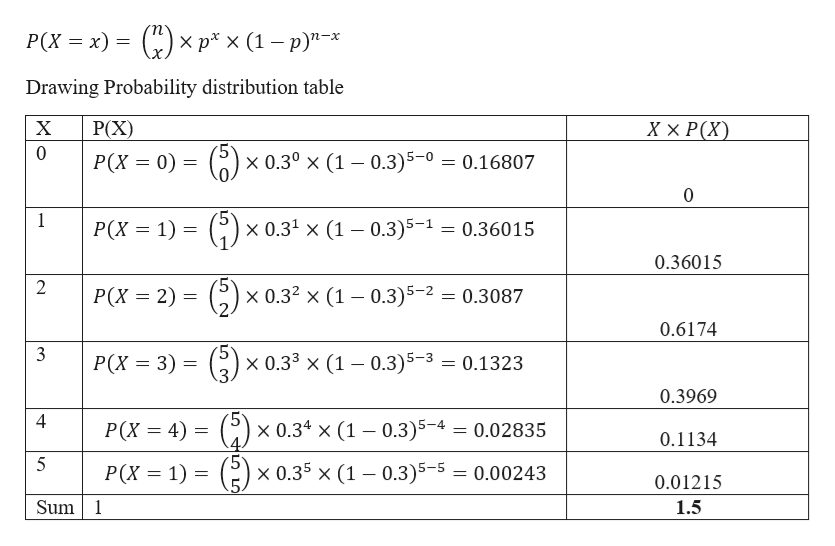# FInd the expected number of infected ducks in a sample of 5 ducks if the probability of an infected duck is .3 and the ducks are considered to be independent.

Question
18 views

FInd the expected number of infected ducks in a sample of 5 ducks if the probability of an infected duck is .3 and the ducks are considered to be independent.

check_circle

Step 1

Given data

No of ducks = 5

Probability of an infected duck p = 0.3

Using Binomial theorem

Probability of x success out of n trial is given byhelp_outlineImage Transcriptionclose(К)x р*x (1—р)"-* Р(х %3 х) — Drawing Probability distribution table XX Р(X) X Р(X) 0 Р(x — 0) — x 0.30 x (1 0.3)5-0 0.16807 0 1 Р(x — 1) — x 0.31 x (1 -0.3)5-1 = 0.36015 0.36015 2 x 0.32 x (1 0.3)5-2 Р(x 3 2) — 0.3087 0.6174 3 Р(X — 3) - x 0.33 x (1 -0.3) 5 -3 0.1323 0.3969 4 Р(x 3D 4) 3D (С) x 0.34 x (1 -0.3)5-4 0.02835 0.1134 5 P(X 1) ( x 0.35 x (1 -0.3)5-5 0.00243 0.01215 Sum1 1.5 fullscreen
Step 2

Expected number of infected ducks in a...

### Want to see the full answer?

See Solution

#### Want to see this answer and more?

Solutions are written by subject experts who are available 24/7. Questions are typically answered within 1 hour.*

See Solution
*Response times may vary by subject and question.
Tagged in

### Basic Probability